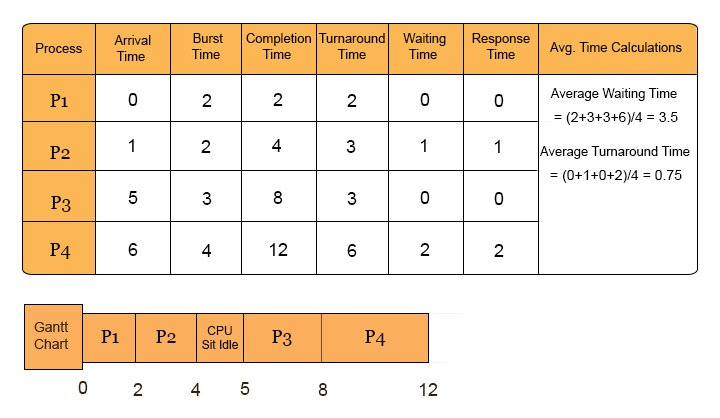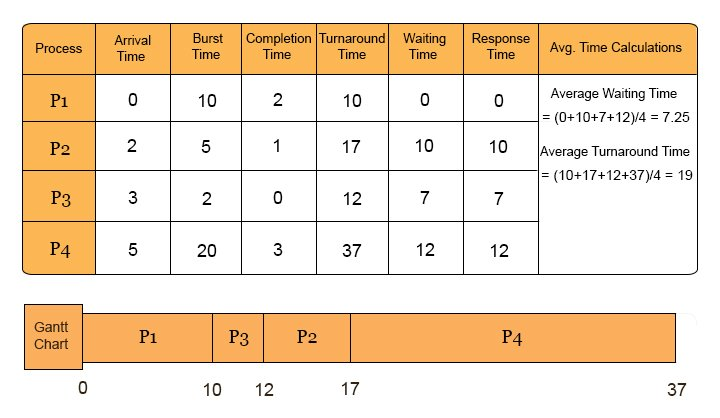Select Page

# Non-Preemptive Scheduling Algorithms

## Formulas

• Completion Time = Time at which Process terminate (see at gantt chart)
• Turnaround Time = Completion time- Arrival time    OR    waiting time+ Burst Time
• Waiting Time = Turnaround time – Burst Time
• Response Time = In Non-Preemptive Response Time= Waiting time
• Response Ratio = (waiting_time + service_time) / service_time
• Avg. Turnaround Time = Sum of turnaround time of all processes/ total processes

Let’s explain non preemptive scheduling algorithms with examples,

In every example Given Fields are

• Process No
• Burst Time
• Burst Time

We have to find the following fields:

• Completion Time
• Turnaround Time
• Waiting Time
• Response Time
• Average Turnaround Time

## 1. First Come First Serve (FCFS)

• It Depends on Arrival Time of process## 2. Shortest Job First (SJF)

It depends on Burst Time of process.

Note: If burst time is the same for two processes then check their the arrival time. if arrival time is also same then check their the process ID. Any process having less burst time or arrival time or process ID is executed first.

## 3. Largest Job First (LJF)

It can also be treated as the same concept of Shortest Job first (SJF) except the Burst time.IF Burst time is higher in LJF then that process will execute first.But in  SJF if the process having the less Burst Time process will execute first.

## 4. Highest Priority (0 is the highest priority)

• Low value (0) can be considered as Highest Priority or lower Priority to choose a process for execution. In this example we will considered Zero as the Highest Priority.## 5. Highest response ratio Next (HRRN)

• Highest Response Ratio Process are executed First

Explanation

As

• Response Ratio = (waiting_time + service_time) / service_time
• Waiting time= Time at which Process get the CPU first time- Arrival time
• Service Time: Service time is the Burst Time

P1 is executed first because at 0 time no other process is arrive except P1. Only P2 arrive while execution of P1.So execution of P2 will alter, after P1.

While execution of P2 Processes P3, P4 and P5 are arrived. So, Check Response Ratio at time point 9 in Gantt chart of Processes P3, P4 and P5

• P3 Responce Ratio = (5+4)/4= 2.25
• P4 Responce Ratio = (3+5)/5= 1.6
• P5 Responce Ratio = (1+2)/2= 1.5

As P3 Having the Highest Response ratio. So, it will execute first.

As P3 complete its execution at time 13. No other process arrive in this time. So, Check Responce Ratio at time 13 in Gantt. Chart for remaining processes P4 and P5.

• Responce Ratio of P4 = (7+5)/5= 2.4
• Responce Ratio of P5 = (5+2)/2= 3.5

As P5 having highest Response Ratio. So, P5 executed first. In the end, P4 is executed because no other process remaining to comparison for HRRN. In this way, all the processes are executed successfully through Highest Response ratio.

Note: Waiting time of A is 5 at time 9 in Gantt Chart because it comes at time 4 and gets CPU at 9 so waiting time = 9-4=5

All Preemptive scheduling algorithms are explained in the next lecture.

Help Other’s By Sharing…